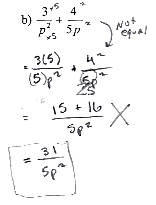Categories

# Squaring doesn’t make equivalent fractionsSquaring doesn’t make equivalent fractions.

Thanks again to Gregory Taylor for the submission.

## 2 replies on “Squaring doesn’t make equivalent fractions”

I don’t know a silver bullet for getting students to resist the temptation of “do a common operation to make this expression look more amenable, even if it changes the expression”.

I like the comment that the fractions are not equivalent. I doubt it would help, but I like to remind students that one reason the operation

a/b + c/d = (a+c)/(b+d)

cannot be right is that, for instance, if you add using this operation

1/2 + 2/3 = 3/5

and separately

2/4 + 2/3 = 4/7

you get two inequivalent fractions even though we added the same rational numbers in both cases. I don’t see a good way to link this sort of observation directly to the mistake above.

We all make mistakes sometimes. How about checking with some easy values (like p = 1 for sure, and maybe p = 2) to notice that something’s wrong and then possibly fix it?